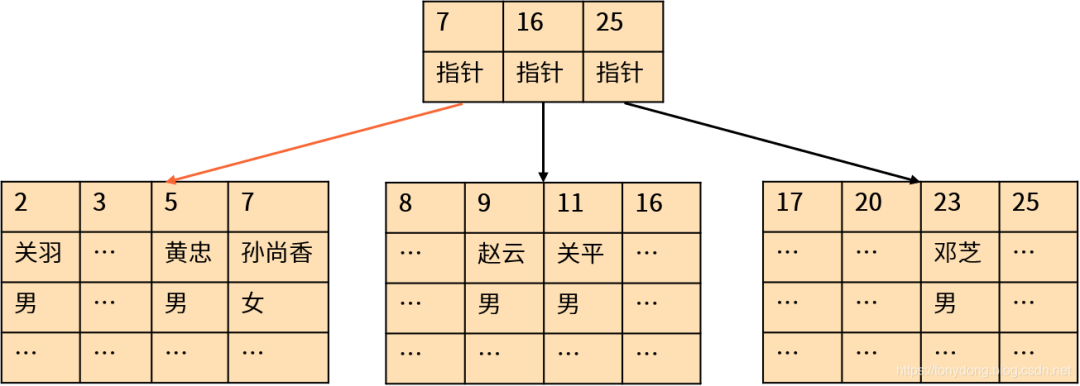## 只返回需要的结果

`一定要为查询语句指定 WHERE 条件，过滤掉不需要的数据行。`通常来说，OLTP 系统每次只需要从大量数据中返回很少的几条记录；指定查询条件可以帮助我们通过索引返回结果，而不是全表扫描。绝大多数情况下使用索引时的性能更好，因为索引（B-树、B+树、B*树）执行的是二进制搜索，具有对数时间复杂度，而不是线性时间复杂度。以下是 MySQL 聚簇索引的示意图：Sql优化

## 确保查询使用了正确的索引

• `经常出现在 WHERE 条件中的字段建立索引`可以避免全表扫描；
• `将 ORDER BY 排序的字段加入到索引中`，可以避免额外的排序操作；
• `多表连接查询的关联字段建立索引`，可以提高连接查询的性能；
• `将 GROUP BY 分组操作字段加入到索引中`，可以利用索引完成分组。 即使创建了合适的索引，如果 SQL 语句写的有问题，数据库也不会使用索引。导致索引失效的常见问题包括：
• 在 WHERE 子句中`对索引字段进行表达式运算或者使用函数都会导致索引失效`，这种情况还`包括字段的数据类型不匹配`，例如字符串和整数进行比较；
• `使用 LIKE 匹配时，如果通配符出现在左侧无法使用索引`。对于大型文本数据的模糊匹配，应该考虑数据库提供的全文检索功能，甚至专门的全文搜索引擎（Elasticsearch 等）；
• `如果 WHERE 条件中的字段上创建了索引，尽量设置为 NOT NULL`；不是所有数据库使用 IS [NOT] NULL 判断时都可以利用索引。 执行计划（execution plan，也叫查询计划或者解释计划）是数据库执行 SQL 语句的具体步骤，例如通过索引还是全表扫描访问表中的数据，连接查询的实现方式和连接的顺序等。如果 SQL 语句性能不够理想，我们首先应该查看它的执行计划，`通过执行计划（EXPLAIN）确保查询使用了正确的索引。`

## 尽量避免使用子查询

 `123456789101112` ``````EXPLAIN ANALYZE SELECT emp_id, emp_name FROM employee e WHERE salary > ( SELECT AVG(salary) FROM employee WHERE dept_id = e.dept_id); -> Filter: (e.salary > (select #2)) (cost=2.75 rows=25) (actual time=0.232..4.401 rows=6 loops=1) -> Table scan on e (cost=2.75 rows=25) (actual time=0.099..0.190 rows=25 loops=1) -> Select #2 (subquery in condition; dependent) -> Aggregate: avg(employee.salary) (actual time=0.147..0.149 rows=1 loops=25) -> Index lookup on employee using idx_emp_dept (dept_id=e.dept_id) (cost=1.12 rows=5) (actual time=0.068..0.104 rows=7 loops=25) ``````

 `123456789101112131415` ``````EXPLAIN ANALYZE SELECT e.emp_id, e.emp_name FROM employee e JOIN (SELECT dept_id, AVG(salary) AS dept_average FROM employee GROUP BY dept_id) t ON e.dept_id = t.dept_id WHERE e.salary > t.dept_average; -> Nested loop inner join (actual time=0.722..2.354 rows=6 loops=1) -> Table scan on e (cost=2.75 rows=25) (actual time=0.096..0.205 rows=25 loops=1) -> Filter: (e.salary > t.dept_average) (actual time=0.068..0.076 rows=0 loops=25) -> Index lookup on t using (dept_id=e.dept_id) (actual time=0.011..0.015 rows=1 loops=25) -> Materialize (actual time=0.048..0.057 rows=1 loops=25) -> Group aggregate: avg(employee.salary) (actual time=0.228..0.510 rows=5 loops=1) -> Index scan on employee using idx_emp_dept (cost=2.75 rows=25) (actual time=0.181..0.348 rows=25 loops=1) ``````

## 不要使用 OFFSET 实现分页

Sql优化

 `12345` ``````-- MySQL SELECT * FROM large_table ORDER BY id LIMIT 10 OFFSET N; ``````

 `123456` ``````-- MySQL SELECT * FROM large_table WHERE id > last_id ORDER BY id LIMIT 10; ``````

## 了解 SQL 子句的逻辑执行顺序

 `12345678910` ``````(6)SELECT [DISTINCT | ALL] col1, col2, agg_func(col3) AS alias (1) FROM t1 JOIN t2 (2) ON (join_conditions) (3) WHERE where_conditions (4) GROUP BY col1, col2 (5)HAVING having_condition (7) UNION [ALL] ... (8) ORDER BY col1 ASC,col2 DESC (9)OFFSET m ROWS FETCH NEXT num_rows ROWS ONLY; ``````

1. 首先，`FROM 和 JOIN 是 SQL 语句执行的第一步`。它们的逻辑结果是一个笛卡尔积，决定了接下来要操作的数据集。注意逻辑执行顺序并不代表物理执行顺序，实际上数据库在获取表中的数据之前会使用 ON 和 WHERE 过滤条件进行优化访问；
2. 其次，`应用 ON 条件对上一步的结果进行过滤并生成新的数据集`
3. 然后，`执行 WHERE 子句对上一步的数据集再次进行过滤`。WHERE 和 ON 大多数情况下的效果相同，但是外连接查询有所区别，我们将会在下文给出示例；
4. 接着，`基于 GROUP BY 子句指定的表达式进行分组`；同时，对于每个分组计算聚合函数 agg_func 的结果。经过 GROUP BY 处理之后，数据集的结构就发生了变化，只保留了分组字段和聚合函数的结果；
5. 如果存在 GROUP BY 子句，可以`利用 HAVING 针对分组后的结果进一步进行过滤`，通常是针对聚合函数的结果进行过滤；
6. 接下来，`SELECT 可以指定要返回的列`；如果指定了 DISTINCT 关键字，需要对结果集进行去重操作。另外还会为指定了 AS 的字段生成别名；
7. 如果还有`集合操作符`（UNION、INTERSECT、EXCEPT）和其他的 SELECT 语句，执行该查询并且`合并两个结果集`。对于集合操作中的多个 SELECT 语句，数据库通常可以支持并发执行；
8. 然后，`应用 ORDER BY 子句对结果进行排序`。如果存在 GROUP BY 子句或者 DISTINCT 关键字，只能使用分组字段和聚合函数进行排序；否则，可以使用 FROM 和 JOIN 表中的任何字段排序；
9. 最后，`OFFSET 和 FETCH（LIMIT、TOP）限定了最终返回的行数`。 了解 SQL 逻辑执行顺序可以帮助我们进行 SQL 优化。例如 WHERE 子句在 HAVING 子句之前执行，因此我们应该尽量使用 WHERE 进行数据过滤，避免无谓的操作；除非业务需要针对聚合函数的结果进行过滤。

 `1234` ``````-- 错误示例 SELECT emp_name AS empname FROM employee WHERE empname ='张飞'; ``````

 `1234` ``````-- GROUP BY 错误示例 SELECT dept_id, emp_name, AVG(salary) FROM employee GROUP BY dept_id; ``````

 `123456789101112131415161718` ``````SELECT e.emp_name, d.dept_name FROM employee e LEFT JOIN department d ON (e.dept_id = d.dept_id) WHERE e.emp_name ='张飞'; emp_name|dept_name| --------|---------| 张飞 |行政管理部| SELECT e.emp_name, d.dept_name FROM employee e LEFT JOIN department d ON (e.dept_id = d.dept_id AND e.emp_name ='张飞'); emp_name|dept_name| --------|---------| 刘备 | [NULL]| 关羽 | [NULL]| 张飞 |行政管理部| 诸葛亮 | [NULL]| ... ``````

## 总结

SQL 优化本质上是了解优化器的的工作原理，并且为此创建合适的索引和正确的语句；同时，当优化器不够智能的时候，手动让它智能。